# $$\ECCVMETHODNAME$$: Correspondence Distillation from NeRF-based GAN

arXiv, 2022

## Abstract

The neural radiance field (NeRF) has shown promising results in preserving the fine details of objects and scenes. However, unlike mesh-based representations, it remains an open problem to build dense correspondences across different NeRFs of the same category, which is essential in many downstream tasks. The main difficulties of this problem lie in the implicit nature of NeRF and the lack of ground-truth correspondence annotations. In this paper, we show it is possible to bypass these challenges by leveraging the rich semantics and structural priors encapsulated in a pre-trained NeRF-based GAN. Specifically, we exploit such priors from three aspects, namely 1) a dual deformation field that takes latent codes as global structural indicators, 2) a learning objective that regards generator features as geometric-aware local descriptors, and 3) a source of infinite object-specific NeRF samples. Our experiments demonstrate that such priors lead to 3D dense correspondence that is accurate, smooth, and robust. We also show that established dense correspondence across NeRFs can effectively enable many NeRF-based downstream applications such as texture transfer.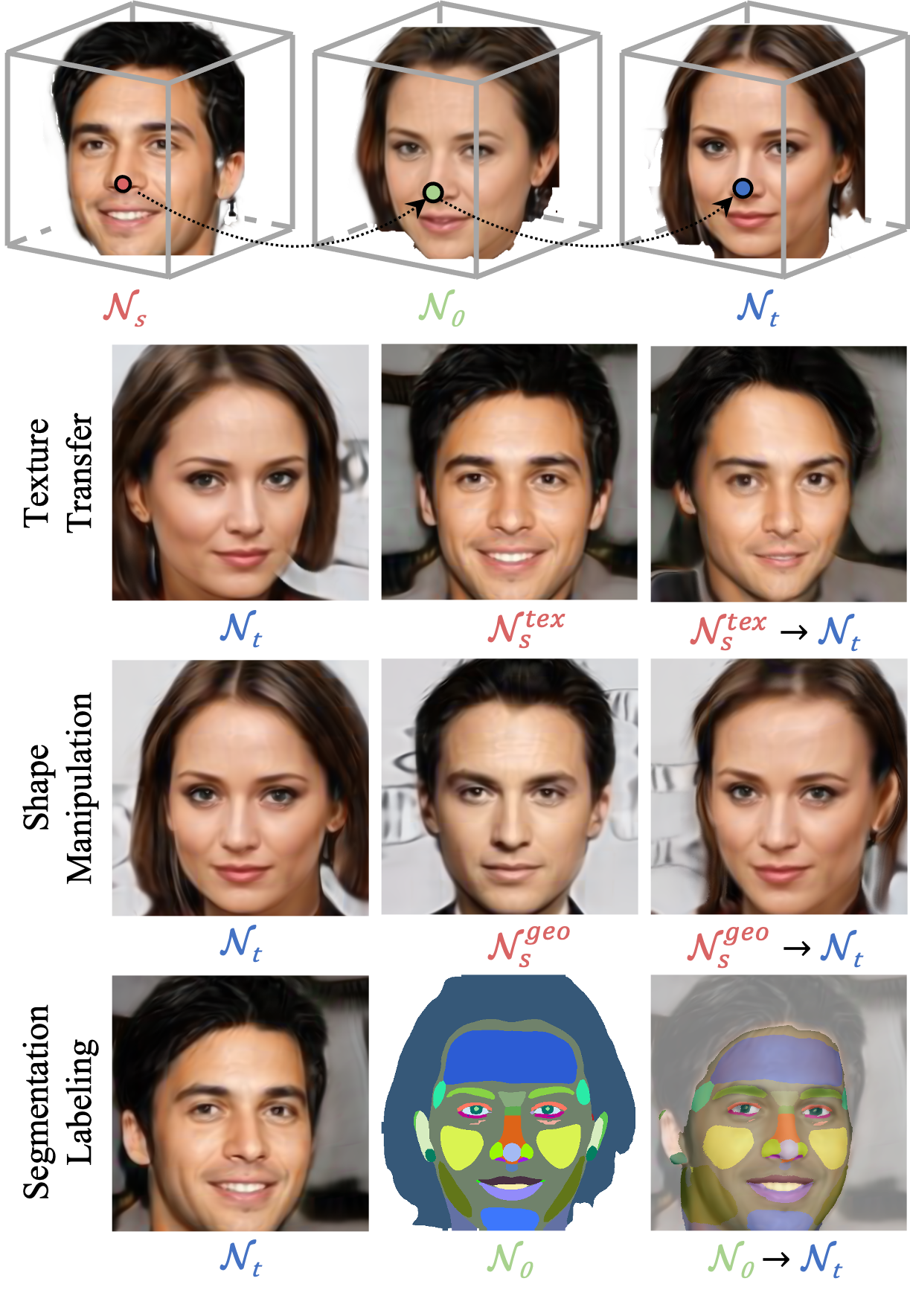We present a novel way to distill dense NeRF correspondence from a pre-trained NeRF GAN in an unsupervised manner.

## for plausible shape inversion

The triple role of a NeRF-based GAN: We retrofit a pretrained NeRF-based GAN into triple roles: (1) the latent codes $$z_{i=1}^{N}$$ serve as holistic structure descriptors; (2) the extracted generator features serve as geometry-aware local descriptors; and (3) the sampling space of pretrained $$G$$ could serve as an infinite object-specific dataset.

## Pipeline

Overview of the proposed Dual Deformation Field $$\ECCVMETHODNAME$$. (a) $$\ECCVMETHODNAME$$ consists of two coordinate-based deformation fields, namely the backward $$B$$ and the forward $$F$$. To get the correspondence point given a point $$\spoint$$ sampled from the source NeRF $$\nerf_s$$, the $$B$$ model conditions on the $$\code_s$$ and learns to deform the input point $$\spoint$$ to the correspondence point in the template NeRF $$\nerf_0$$. Similarly, the $$F$$ model conditions on the target latent code $$\code_t$$ and learns to deform points from the template NeRF $$\point_0$$ to the target NeRF $$\nerf_t$$. (b): Feature similarity losses $$\mathcal{L}^B_{sim}$$ and $$\mathcal{L}^F_{sim}$$ between features extracted from the generator of the pre-trained $$\pi$$-GAN, $$G$$, is adopted as the main loss.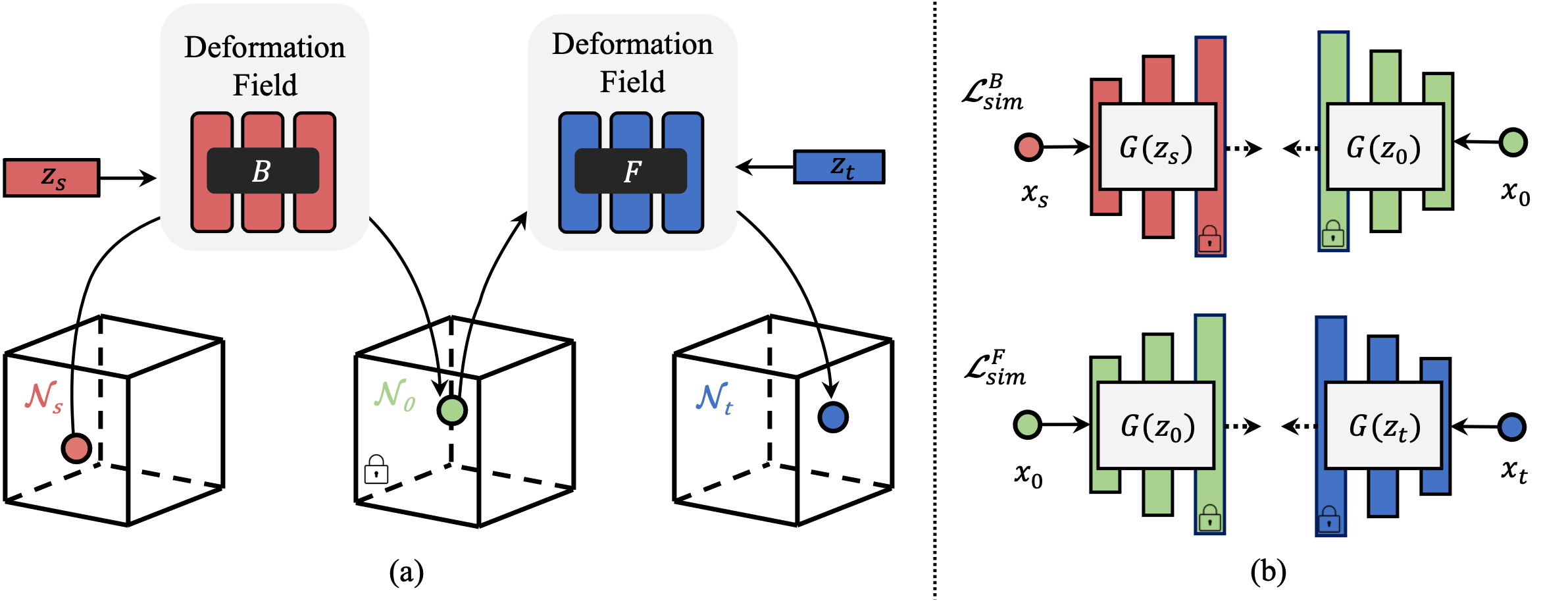## for High-quality editing

Illustration of loss functions used in $$\ECCVMETHODNAME{}$$. (a) Backward cycle-consistency loss: $$F(B(\spoint, \code_s), \code_s) \approx \spoint$$, (b) forward cycle-consistency loss: $$(F(\tpoint, \code_t), \code_t) \approx \tpoint$$ and (c) second-order feature similarity loss: $$G(\spoint, \code_s) \approx G(F(B(\spoint, \code_s),\code_t),\code_t)$$.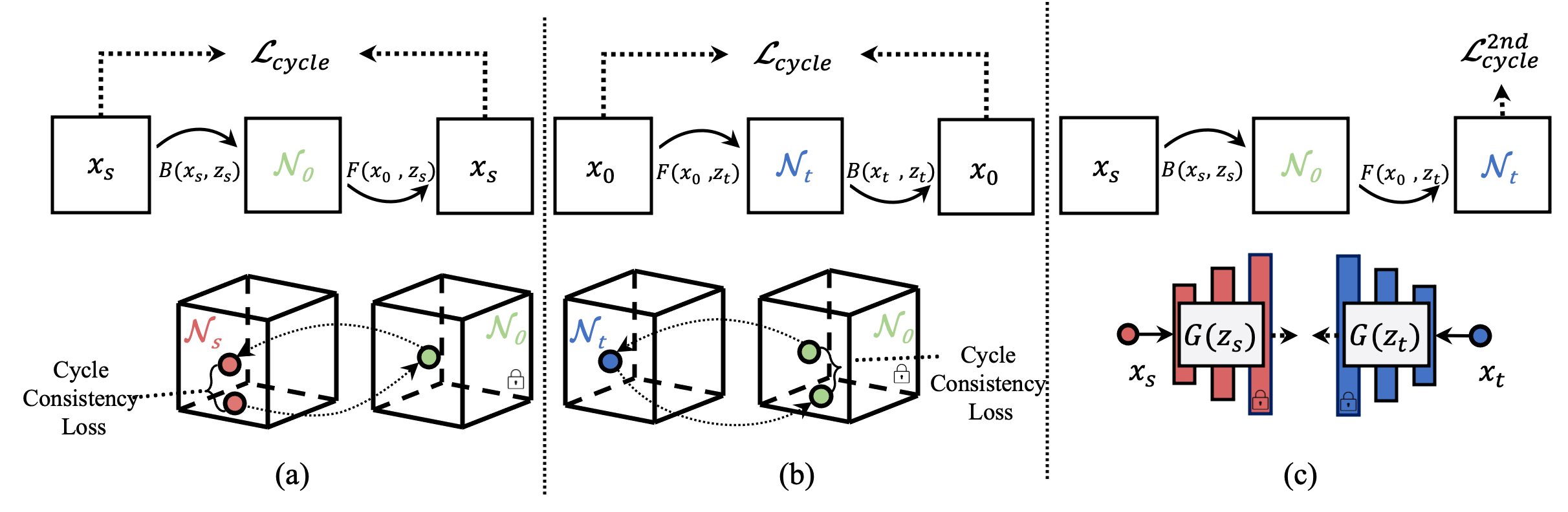## Transfer

Through dense 3D correspondence established via $$\ECCVMETHODNAME$$, our method could conduct texture transfer over real worl identities. Visually inspected, our method produces semantic plausible dense correspondences with high-fidelity texture transfer results. We show our results in multiple views to demonstrate that our method has learned both 3D consistent dense correspondences. Note that good texture transfer results could not be achieved without accurate correspondence matching in 3D space.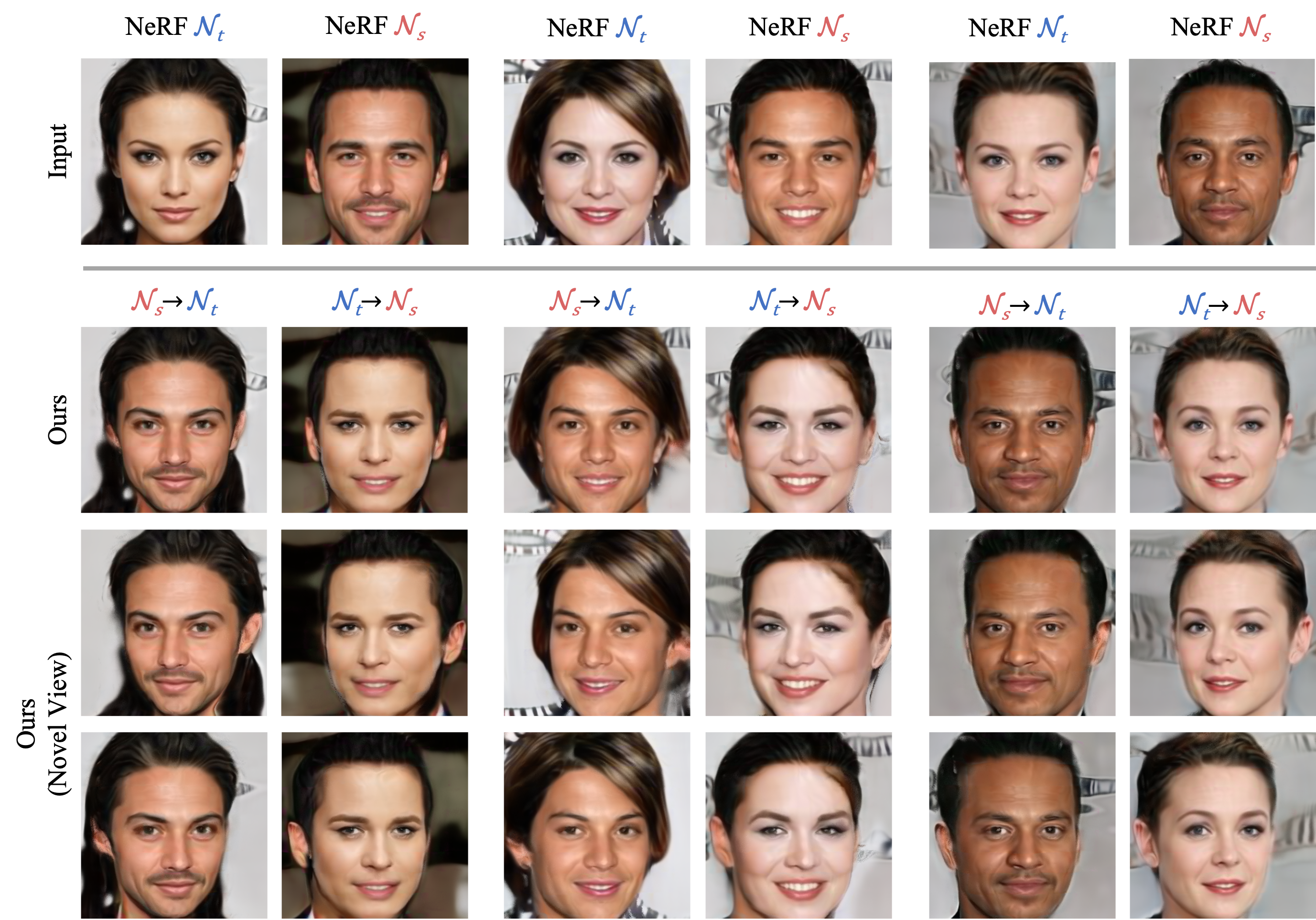## Transfer

Given a single canonical segmentation map, $$\ECCVMETHODNAME$$ conducts 1-shot segmentation transfer with high quality. The segmentation results are both accurate and also view-consistent.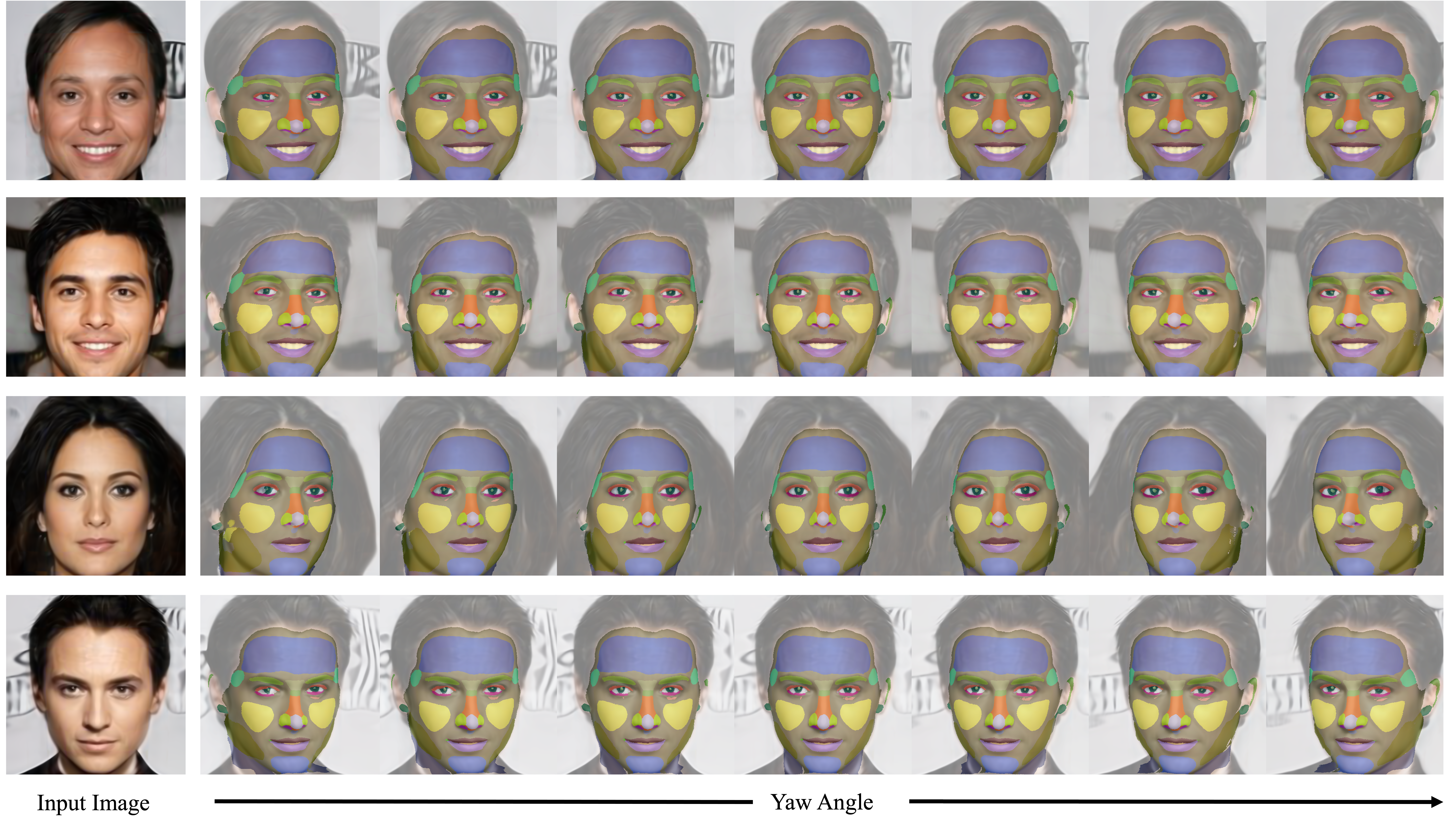We design a hybrid inversion framework to allow $$\ECCVMETHODNAME$$ to establish 3D correspondence over real identities and facilitate downstream tasks like view synthesis, segmentation transfer and shape editings.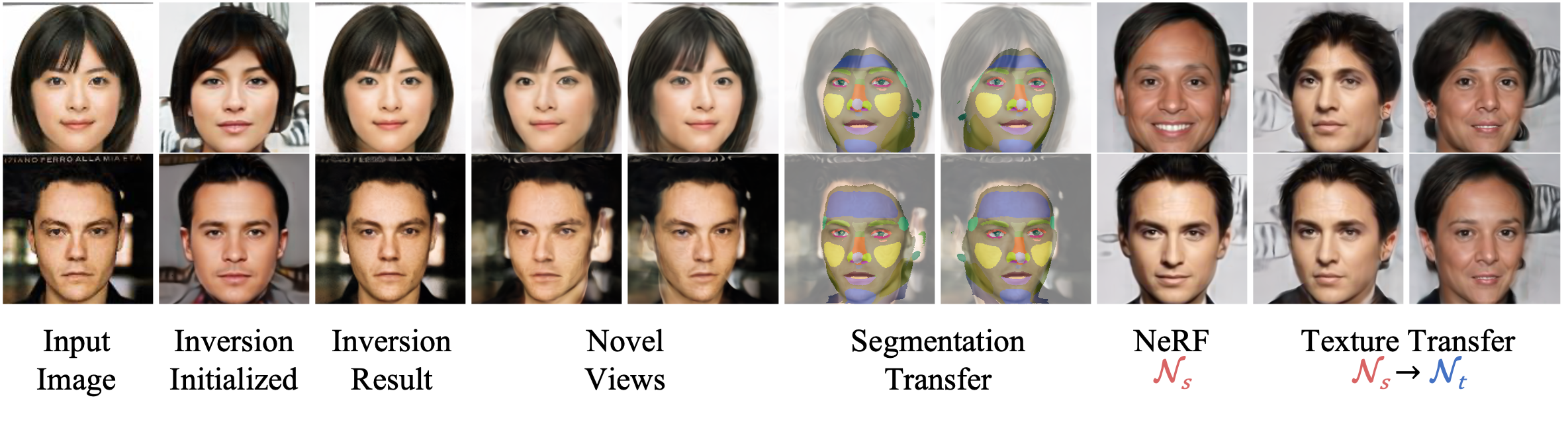## Citation

@article{lan2022ddf,
title={DDF: Correspondence Distillation from NeRF-based GAN},
author={Lan, Yushi and Loy, Chen Change and Dai, Bo},
journal={arXiv preprint arXiv:2212.09735},
year={2022}
}

## Projects

• Self-supervised Geometry-Aware Encoder for Style-based 3D GAN
Y. Lan, C. C. Loy, B. Dai
Technical report, arXiv:2212.07409, 2023
[arXiv]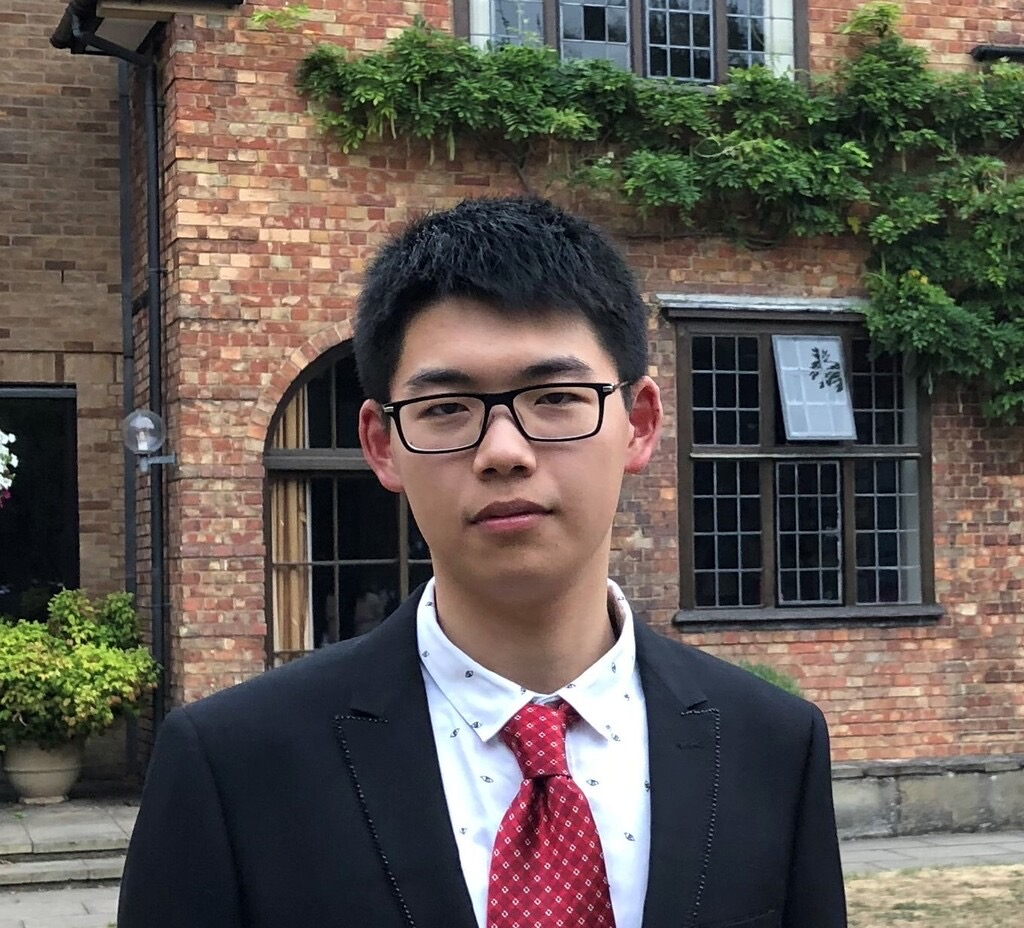Contact

Yushi Lan
Email: yushi001 at e.ntu.edu.sg